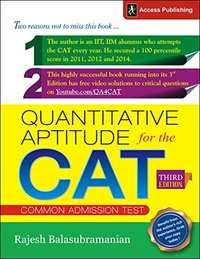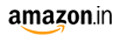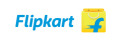# Quantitative Aptitude for the CAT CATM.R.P 595/-194.00(67%)535.00(10%)
Product Description

Key Features:

• 27 core Chapters
• Each chapter contains theory, solved examples and exercises based on difficulty levels
• All questions with detailed solutions
• Difficulty levels indicated along with solutions
• Question papers of Quantitative Aptitude of 2006, 2007 & 2008 with solutions for first two
• Three high quality Mock CAT papers on Quantitative Aptitude with solutions
• A new Final Mock CAT (Quants ) Paper at the end for a final assessment of preparation level

Six broad preparation strategies in this book:

• Carefully graded difficulty level of questions
• Each question has detailed solution
• Solutions supported by relevant thought-process
• Focus on 'best method approach' to each question
• Each topic ends with a set of CAT questions ( difficulty levels 1 & 2)
• How to prepare intensely, yet enjoy the preparation

• Articles on How to Prepare for the CAT
• Article-1: Coping With Pressure
• Article-2: Common Traps To Avoid In The Quant Section
• Article-3: What Should You Make Right This CAT Vis-a-Vis Your Last CAT?
• Article-5: Under What Circumstances Is It A Good Idea To Quit Your Job And Prepare For The CAT?
• Previous Years' CAT Papers and Mock Test Papers (Quants)
• Solved CAT (Quants) 2006
• Solved CAT (Quants) 2007
• Unsolved CAT (Quants) 2008
• Solved Mock-1 (Quants)
• Solutions
• Solved Mock-2 (Quants)
• Solutions
• Unsolved Mock-3 (Quants)

1. Averages

• Exercise Problems
• CAT level Questions
• Solutions

2. Percentages

• Percentages and Fractions
• Decimals and Percentages
• Exercise Problems
• CAT level Questions
• Solutions

3. Profit and Loss

• Some Important Terms
• Marked Price and Discount
• Exercise Problems
• CAT level Questions
• Solutions

4. Linear Equations

• Unique Solution, Infinite Solutions and No Solution
• Linear Equations - Norms for Solutions
• Exercise Problems
• CAT level Questions
• Solutions
• Solving Quadratic Equations - Usually by Splitting the Middle Term
• Guidelines for Number/Type of Roots
• Sum and Product of Roots
• Exercise Problems
• CAT level Questions
• Solutions
6. Ratio, Proportion, Variation Ratios
• Comparison of Ratios
• Proportions
• Variations
• Exercise Problems
• CAT level Questions
• Solutions
7. Mixtures
• Exercise Problems
• CAT level Questions
• Solutions
8. Speed, Time and Distance
• Keeping an Eye on the Units
• Quantitative Aptitude for the CAT
• Understanding the Formula
• The Idea of Average Speed
• The Idea of Relative Speed
• Ideas with Trains and Boats
• Exercise Problems
• CAT level Questions
• Solutions
9. Races
• Race along a Straight Track
• Circular Race
• Exercise Problems
• CAT level Questions
• Solutions
10. Pipes and Cisterns
• Rate of Flow
• Exercise Problems
• CAT level Questions
• Solutions
11. Progressions
• Arithmetic Progression
• Properties of an A.P.
• Geometric Progression
• Properties of a G.P.
• Harmonic Progressions
• Properties of H.P.
• A.M.-G.M.-H.M. Inequality
• Exercise Problems
• CAT level Questions
• Solutions
12. Simple Interest and Compound Interest
• Compound Interest
• Different compounding periods
• Time Taken for a Sum of Money to Double - (in Compound Interest)
• Simple and Compound Interest - Some Key Points
• Exercise Problems
• CAT level Questions
• Solutions
13. Exponents and Logarithms
• Basic Properties of Logarithms
• Properties of Exponents
• Properties of Logarithms
• A few more Properties in Logarithms
• Characteristic and Mantissa
• Inequalities in Logarithms
• Exercise Problems
• CAT level Questions
• Solutions
14. Binomial Theorem
• Some Key Properties of Binomial Expansion
• Properties of nCr for Simplification
• Exercise Problems
• CAT level Questions
• Solutions
15. Clocks
• Key Idea - How Much does the Minute Hand ‘Gain'? Other Key Points
• How Many Times do the Minute and Hour Hands Coincide?
• 180° and 90° Angles
• Exercise Problems
• CAT level Questions
• Solutions
> 16. Calendars
• Calendars
• Exercise Problems
• CAT level Questions
• Solutions
• Quantitative Aptitude for the CAT
17. Inequalities
• Do's: List of Items We can do while Solving Inequalities
• List of Items We should avoid while Solving Inequalities
• Dealing with - x
• Number Line
• Range, Open Interval, Closed Interval
• Role of +, -
• Idea of between the Roots and Outside the Roots
• Inequalities with Modulus
• AM- GM Inequality
• Inequalities in Exponents and Logarithms
• Exercise Problems
• CAT level Questions
• Solutions
18. Functions
• Domain and Range of a Function
• Even and Odd Functions
• Properties of Even Functions and Odd Functions
• Surjective, Injective and Bijective Functions
• Bijective function
• Monotonically Increasing, Decreasing, Non-inc reasing and Non-decreasing
• Exercise Problems
• CAT level Questions
• Solutions
19. Set Theory
• Types of Sets
• Union and Intersection of Two Sets
• Key Property with Cardinal Number
• Properties with Three Sets
• Exercise Problems
• CAT level Questions
• Solutions
> 20. Number Theory
• Number Classifications
• Some Interesting Properties of Prime Numbers
• Properties of Odd and Even Numbers
• Factors and Multiples
• Factorisation, Prime Factorisation and the Fundamental Theorem of Arithmetic HCF and LCM
• HCF - Highest Common Factor
• LCM - Least Common Multiple
• Coprime Numbers
• Fractions
• Decimals
• Rules of Exponents
• Fibonacci Numbers
• Prime Factorisations-Some Simple Properties
• Factors, Properties
• Rationale Behind the Above Formulae
• Remainders: Three Key Ideas
• First Idea - Definition
• Second Idea - Remainders are Consistent for Addition, Subtraction, and Multiplication
• Third Idea-Remainders Follow a Pattern
• Fermat's Little Theorem
• Euler's Function
• Wilson's Theorem
• Factorial
• Remainders on Successive Division
• Expressing Number with Different Base
• Converting from Decimal to Some Other Base
• Base n Mathematic
• Exercise Problems
• CAT level Questions
• Solutions
21. Polynomials
• Simple Expansions and Factorisations
• Some Other Key Expansions
• Polynomial Remainder Theorem
• Partial Fraction Decomposition
• Factorisation Properties
• Rules for Sum/Product of Roots for Higher Order Polynomials
• Exercise Problems
• CAT level Questions
• Solutions
• Quantitative Aptitude for the CAT
22. Combinatorics
• Key Concepts and Theory
• Fundamental Rule of Counting
• Rule of Sum
• The Idea of Order
• The Idea of Repetition
• Rearrangements
• Problems Based on Digits of A Number
• Problems Based on Rearrangement of Letters of A Word
• Problems Based on Dice
• Problems Based on Coin Tosses
• Problems Based on Card Packs
• Circular Arrangement
• Selecting One or More from A Set
• Distribution into Groups
• Distribution into Groups - Variants
• Alternative Method Counting and Number Theory
• Exercise Problems
• CAT level Questions
• Solutions
23. Probability
• Deterministic and Probabilistic Events
• Probability of an Event
• Sample Space
• Complementary Events
• Mutually Exclusive Events
• Mutually Exclusive and Collectively Exhaustive Events
• Independent Events
• Exercise Problems
• CAT level Questions
• Solutions
24. Geometry
• Angles
• Types of Angles
• Angles at the Intersection of Lines
• Basic Properties of Lines and Planes
• Parallel Lines and Transversal
• Triangles
• Properties of a Triangle
• Classifications of Triangles
• Pythagoras Theorem and its Extensions
• Angle Bisector Theorem
• Basic Proportionality Theorem
• Congruence
• Similarity
• Centroid, Orthocenter, Incenter, Circumcenter
• Points to Remember
• Equilateral Triangle
• Right-Angled Triangle
• Isosceles Triangle
• Apollonius Theorem
• Two Special Triangles
• Sine Rule, Cosine Rule
• Area of a Triangle (5 Different Formulae)
• Circles
• Definitions
• Basic Properties of a Circle
• Properties of Angles in Circles
• Properties with two Circles
• Properties of Tangents and Secants
• Parallelogram
• Rectangle
• Rhombus
• Square
• Trapezium or Trapezoid
• Kite
• Polygons
• Convex Polygon
• Concave Polygon
• Regular Polygon
• Angle Properties
• Exercise Problems
• CAT level Questions
• Solutions
• Quantitative Aptitude for the CAT
25. Coordinate Geometry
• Section Formula
• Slope of a Line
• Origin Changing Formula
• Line Properties
• Four Ways of Forming a Line Equation
• Slope-point Form
• Two-points Form
• Slope-intercept Form
• Two-intercepts Form
• Parallel Lines and Perpendicular Lines Equation of A Circle
• Exercise Problems
• CAT level Questions
• Solutions
> 26. Trigonometry
• Measures of Angles
• Basic Trigonometric Equations
• Basic Trigonometric Value
• Some Basic Values
• Some Key Results
• Results for Angle Combinations
• Formulae for 2q
• Trigonometric Ratios - Two Key Rules
• Angle of Elevation and Angle of Depression
• Sine Rule, Cosine Rule, Area of Triangle
• Exercise Problems
• CAT level Questions
• Solutions
27. Mensuration
• Two Dimensional Shapes
• Triangle Revisited
• Three-dimensional Shapes
• Exercise Problems
• CAT level Questions
Final Mock-CAT (Quants)
• Solutions
• Content